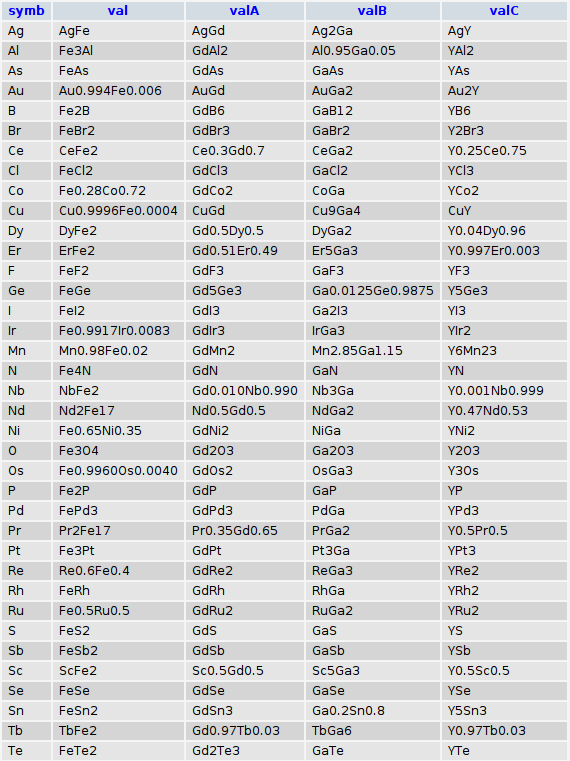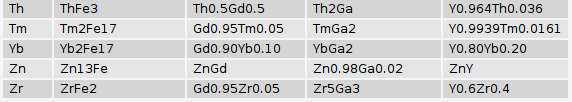# Hex, Bugs and More Physics | Emre S. Tasci

a blog about physics, computation, computational physics and materials…

## (Un)Common?

### June 24, 2008 Posted by Emre S. Tasci

They say that, it is the opposite (unsimilar) couples that make up for much stabler relationships… If you have only 2 different values, (+) and (-), spin up and down, man and woman, then the unsimilar of a thing that is unsimilar to you equals to a thing that is similar to you. Although it is generally not valid for elements, it gives you an insight nonetheless.. So if Y, Gd, Fe and Ga play a similar part in dearest ZrO2‘s life, let’s check for their other common "partners" that are known to have some connection/involvement this or that way…

The table we’re interested in today holds the formula and the constituent elements as in the example below:(Actually the table contains way more properties than the formula and the constituent elements but I’ve focused only to the relevant props)

symbol_A and symbol_B are position oriented wrt the written formula, meaning that for an AxBy binary, you have A for symbol_A and B for symbol_B whereas if it is BxAy, then they are reversed.

We will be looking for elements that form binaries with the aforementioned 4 elements, namely: Yttrium, Gadolinium, Iron and Gallium.

We can search for each of these 4 via the following query and then add it up:

SELECT symbol_A as symb,val1 FROM `dbl014`
WHERE  symbol_B="Gd"
GROUP BY Symbol_A
UNION
SELECT symbol_B as symb, val1 FROM `dbl014`
WHERE (symbol_A="Gd" AND symbol_B!="")
GROUP BY Symbol_B

For the intersection of two elements, we glue the two like the above one via the INNER JOIN function:

SELECT * FROM
(
SELECT symbol_A as symb,val1
FROM `dbl014`
WHERE  symbol_B="Gd"
GROUP BY Symbol_A

UNION

SELECT symbol_B as symb, val1
FROM `dbl014` WHERE (symbol_A="Gd" AND symbol_B!="")
GROUP BY Symbol_B
) AS A

INNER JOIN

(
SELECT symbol_A as symb,val1
FROM `dbl014` WHERE symbol_B="Y"
GROUP BY Symbol_A

UNION

SELECT symbol_B as symb, val1
FROM `dbl014` where (symbol_A="Y" AND symbol_B!="")
GROUP BY Symbol_B
) AS B
USING (symb)
ORDER BY symb

And for the ultimate quattro, it gets even exciting! :

SELECT *
FROM (

SELECT symbol_A AS symb, val1 AS val
FROM `dbl014`
WHERE symbol_B = "Fe"
GROUP BY Symbol_A
UNION
SELECT symbol_B AS symb, val1 AS val
FROM `dbl014`
WHERE (
symbol_A = "Fe"
AND symbol_B != ""
)
GROUP BY Symbol_B
) AS A
INNER JOIN (
SELECT *
FROM (

SELECT symbol_A AS symb, val1 AS valA
FROM `dbl014`
WHERE symbol_B = "Gd"
GROUP BY Symbol_A
UNION
SELECT symbol_B AS symb, val1 AS valA
FROM `dbl014`
WHERE (
symbol_A = "Gd"
AND symbol_B != ""
)
GROUP BY Symbol_B
) AS A
INNER JOIN (
SELECT *
FROM (

SELECT symbol_A AS symb, val1 AS valB
FROM `dbl014`
WHERE symbol_B = "Ga"
GROUP BY Symbol_A
UNION
SELECT symbol_B AS symb, val1 AS valB
FROM `dbl014`
WHERE (
symbol_A = "Ga"
AND symbol_B != ""
)
GROUP BY Symbol_B
) AS A
INNER JOIN (
SELECT symbol_A AS symb, val1 AS valC
FROM `dbl014`
WHERE symbol_B = "Y"
GROUP BY Symbol_A
UNION
SELECT symbol_B AS symb, val1 AS valC
FROM `dbl014`
WHERE (
symbol_A = "Y"
AND symbol_B != ""
)
GROUP BY Symbol_B
) AS B
USING ( symb )
) AS C
USING ( symb )
) AS D
USING ( symb )
ORDER BY symb

and voila!a total of 42 all in all. The "val" columns are there for verificational purposes only – since after applying the GROUP BY function, they all lose their meanings.